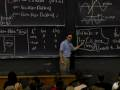# Session 23: Linear Approximation« Previous | Next »

## Overview

Linear approximation is a powerful application of a simple idea. Very small sections of a smooth curve are nearly straight; up close, a curve is very similar to its tangent line. We calculate linear approximations (i.e. equations of tangent lines) near x=0 for some popular functions; we can then change variables to get approximations near x=a.

## Lecture Video and Notes

### Video Excerpts

Flash and JavaScript are required for this feature.» Clip 1: Introduction to Linear Approximation (00:02:00)

From Lecture 9 of 18.01 Single Variable Calculus, Fall 2006

Flash and JavaScript are required for this feature.» Clip 2: Linear Approximation to ln x at x=1 (00:03:00)

From Lecture 9 of 18.01 Single Variable Calculus, Fall 2006

Flash and JavaScript are required for this feature.From Lecture 9 of 18.01 Single Variable Calculus, Fall 2006

Flash and JavaScript are required for this feature.From Lecture 9 of 18.01 Single Variable Calculus, Fall 2006

## Worked Example

Comparing Linear Approximations and Calculator Computations

## Lecture Video and Notes

### Video Excerpts

Flash and JavaScript are required for this feature.From Lecture 9 of 18.01 Single Variable Calculus, Fall 2006

« Previous | Next »